## MODE Function

The MODE function is a premade function in Google Sheets, which is used to find the most common value(s).

There are two versions, which are typed `MODE.SNGL` or `MODE.MULT` that gets a list of cells:

=MODE.SNGL(value1, [value2, ...])
=MODE.MULT(value1, [value2, ...])

`MODE.SNGL` does the same as `MODE` and returns only one value (picking the first it finds).

`MODE.MULT` returns multiple values if there are more than one that is the most common.

Note: The mode is a type of average value, which describes where most of the data is located. You can read more about mode in the Statistics Mode Chapter.

Note: The mode functions work with both text values and numbers.

Let's have a look at some examples!

## Mode Function Example (SNGL)

Find the most common number of all the different Pokeballs in the trainers' inventories.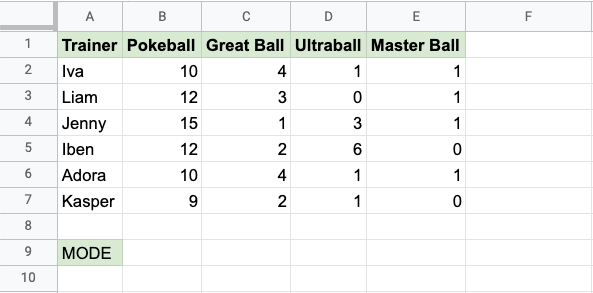Copy Values

The MODE.SNGL function, step by step:

1. Select the cell `B9`
2. Type `=MODE`
3. Click the MODE.SNGL command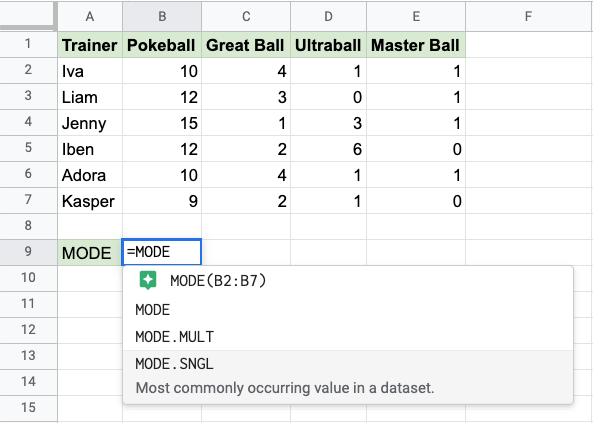1. Specify the range `B2:E7` for all the different types of Pokeballs
2. Hit enter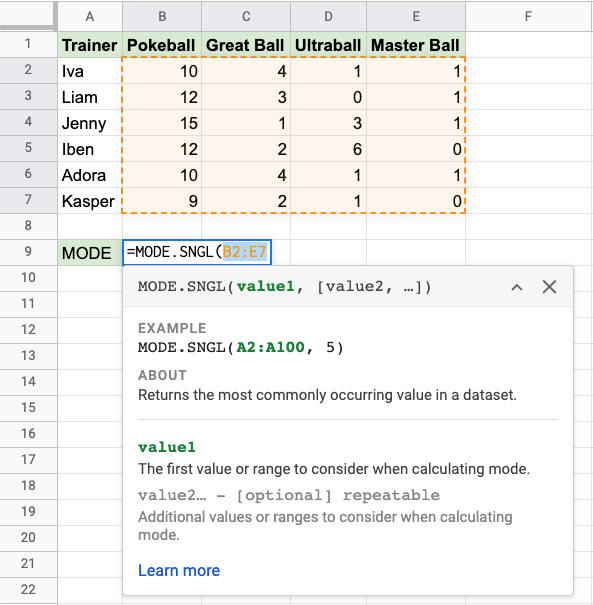Now, the function returns the most common value in the range: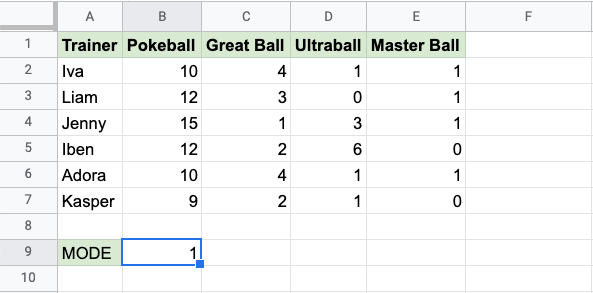It returns the number `1` which is seen 7 times. The closest number to it is `3` and `4` which are seen 3 times each.

## Mode Function Example (MULT)

Find the most common number of Pokeballs in the trainers' inventories.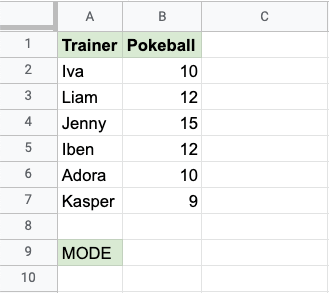Copy Values

The MODE.MULT function, step by step:

1. Select the cell `B9`
2. Type `=MODE`
3. Click the MODE.MULT command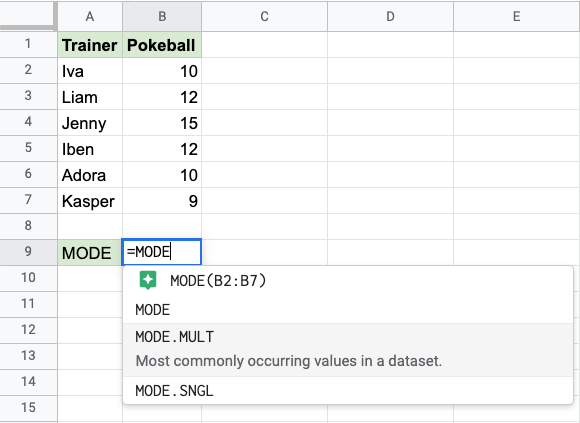1. Specify the range `B2:B7` for the Pokeballs
2. Hit enter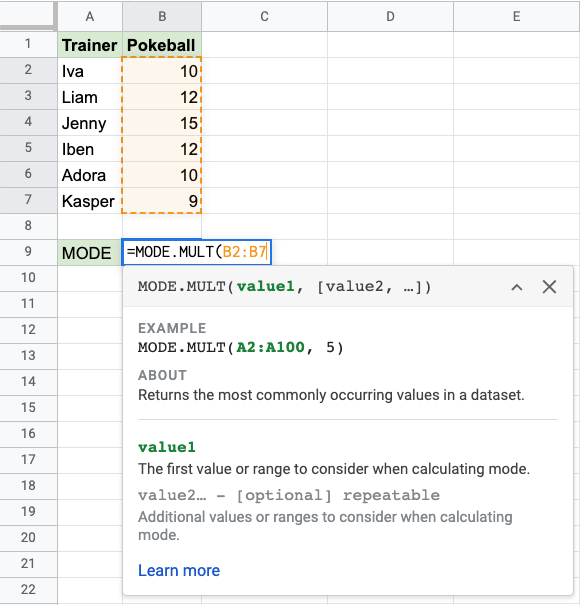Now, the function returns the most common values in the range: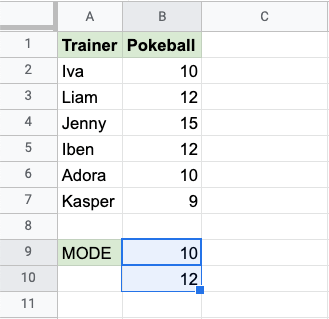It returns the number `10` in the first cell (`B9`) and the number `12` in the second cell (`B10`). Both 10 and 12 are seen twice, which is more than any of the other values.